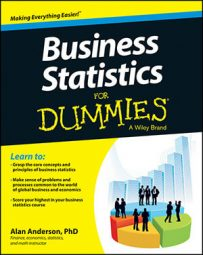Two events are said to be complements if they are mutually exclusive and their union equals the entire sample space. This is represented by the complement rule, which is expressed as follows:

P(AC) = 1 – P(A)

AC is the complement of event A.

Here's an example: Suppose that an experiment consists of choosing a single card from a standard deck. Event A = "the card is red." Event B = "the card is black." Events A and B are complements because A and B are mutually exclusive (no card can be both red and black). The union of A and B is the sample space (the entire deck, because all cards must be either red or black, so the union of A and B equals the entire sample space.)

Joint Probability Distribution for Coffee Styles
Coffee Type Special Reserve Blend (S) Kona Hawaii Blend (K) Aromatic Blend (A) Total
Decaffeinated (D) 0.12 0.80 0.22 0.42
Regular (R) 0.24 0.12 0.22 0.58
Total 0.36 0.20 0.44 1.00

For example, the table shows the distribution of coffees (measured in pounds) the Big Bean Corporation produces during a given day.

The complement of event D (decaffeinated coffee) is event R (regular coffee) because all coffee must be either decaffeinated or regular, and no coffee can be both. You can find the probability of the complement of D as follows:

P(DC) = 1 – P(D)

Referring to the table, you can see that P(D) = 0.42. Therefore, P(DC) = 1 – P(D) = 1 – 0.42 = 0.58, which is equal to P(R).